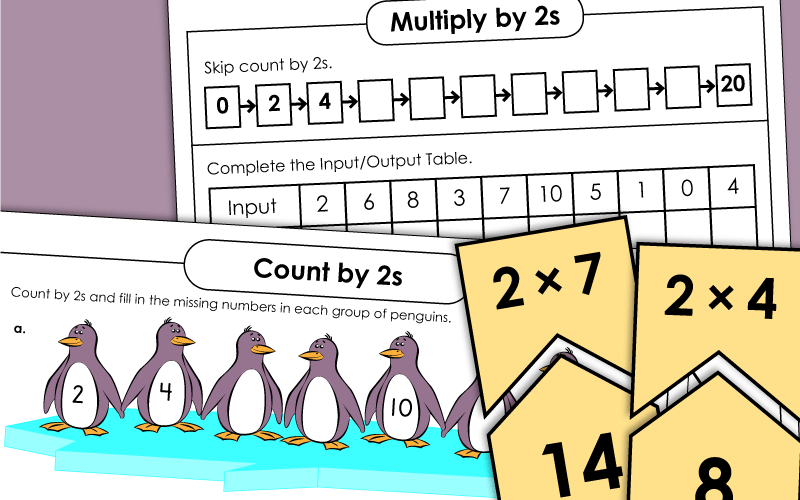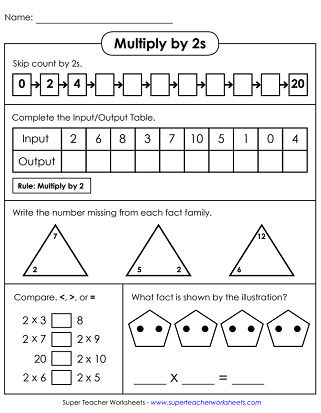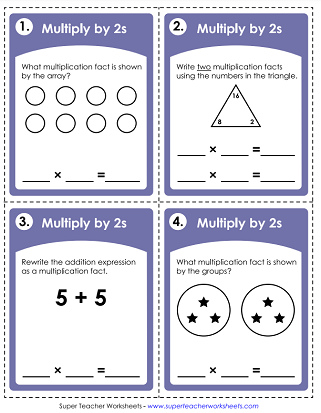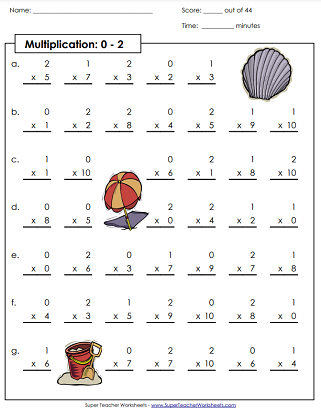# Multiplication by 2s

Print worksheets for teaching students to multiply single-digit numbers by the number 2. This page has printable flash cards, practice worksheets, math sliders, timed tests, puzzles, and task cards.## Multiply By2s Only

Students will learn to multiply by 2s by completing the various activities on this page.
Solve these basic multiplication facts and use the color key at the bottom to reveal a beach scene. Facts are with 0s, 1s, and 2s.
Task cards can be used for classroom scavenger hunts, small group sessions, peer tutoring, exit slips, document cameras, and so much more. These cards have arrays, repeated addition expressions, and fact family triangles.
Cut out the puzzle pieces along the dotted lines and arrange the answers to the correct multiplication facts.
Follow along the caterpillar to complete all of the math facts. Write your answer in the space below the problem.
Cut, color, and fold this origami fortune teller (aka cootie catcher) game.
This file contains flash cards for all basic facts in which 2 is a factor. There's also a sorting mat and a practice quiz.
Print this file on card stock. The cut out the truck and slider strips. Weave the strips through the truck. Slide the strip up and down to see multiplication facts.

## Skip Count by 2s

Practice counting by 2s by counting the fish, flowers, crayons, and snails on this worksheet.
Count by 2s to answer the seven questions on this printable skip-counting worksheet.
Skip count by 2s following along with this number line. Write the missing numbers in the boxes.
Draw a line from dot-to-dot as you count by 2s to reveal a picture of a dolphin.
Use a crayon to color in every second number in this hundreds chart.
Count by 2s and fill in the missing numbers in each group of penguins on this printable multiplication worksheet.
Skip counting is fun when you count by 2s with this worksheet featuring colorful balloons.
Count by 2s in each group of boxes on this martial-arts themed skip counting worksheet.

## All Facts0s through 2s

This worksheet is a multiplication drill to see how fast your students can answer 44 basic multiplication facts correctly. Facts are with 0s, 1s, and 2s multiplied up to 10.
This multiplication drill worksheet is similar to the first version only the factors of 0, 1, and 2 are multiplied up to 12. There are 44 questions to answer on this page.
THis 50 question timed assessment has a mixture of multiplication facts with 0s, 1s, and 2s.
More Multiplication Worksheets

We have basic multiplication worksheets with factors up to 10 or 12. We have multi-digit multiplication pages as well.

Multiplication Tables

Properties of Multiplication

## Sample Worksheet ImagesMy Account
Site Information